# Subtraction 4

Teaching kids additional subtraction strategies is one way to boost maths fluency and comprehension. This guided lesson, designed by our team of curriculum experts, teaches various strategies to find the difference, and provides exercises that help kids to practise these strategies on their own. For more opportunities to practise, try downloading and printing the year four subtraction worksheets recommended by our curriculum team.
This lesson includes 5 printable learning activities.

## Two-Digit Subtraction with Borrowing Mountain Game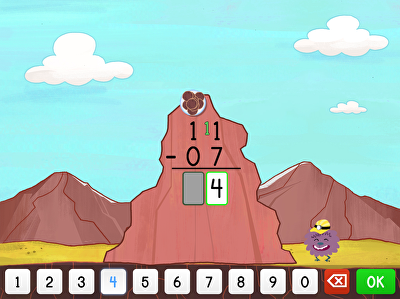Crunch some numbers -- and some mountains -- with this two-digit subtraction with borrowing game that helps third graders scale subtraction. A new and inventive way to practise two-digit subtraction and regrouping online, kids can solve equations and help CuzCuz bust through the mountainside, using rocks to represent the borrowed numbers.

## Two-Digit Subtraction and RegroupingThis exercise showing how to execute two digit subtraction using regrouping makes the second step in subtraction much more accessible.

## Three-Digit Subtraction: Train Edition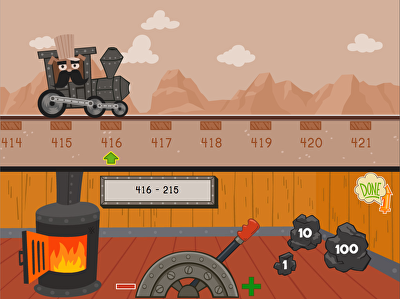All aboard! This train is headin' out, and the conductor needs help to keep it moving. Kids solve three-digit subtraction problems using a number line in this wild west train game. By using coal manipulatives that represent 1, 10, and 100 units, players move the train to land on the difference in as few moves as possible, helping kids strategize the best ways to solve subtraction problems.

## Three-Digit Subtraction on a Number Line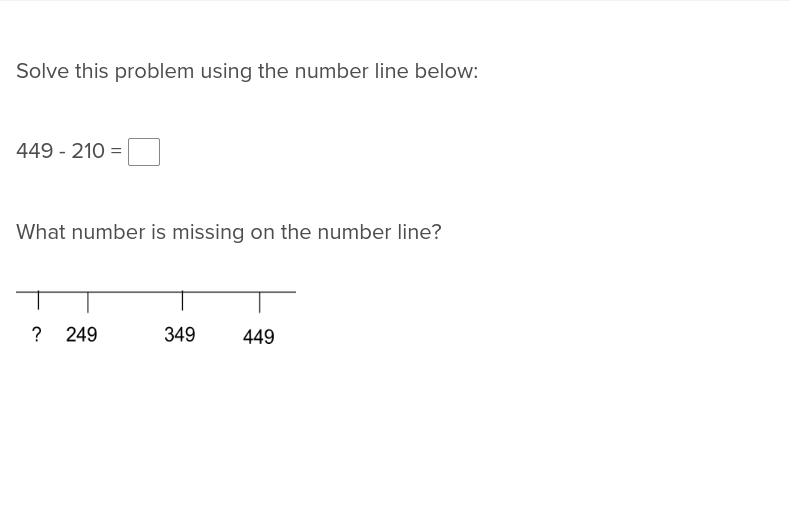Take the difficulty out of three digit subtraction with this exercise that shows students how to reach the correct answer using a number line.

## Estimating to Subtract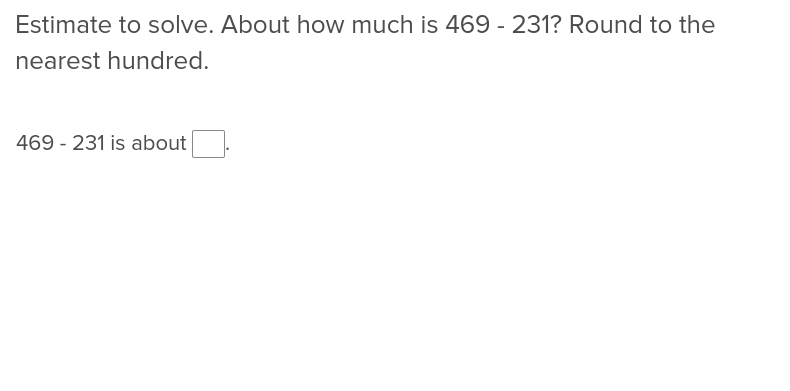For students learning how to estimate during subtraction, this exercise will make matters much simpler.

## Three-Digit Subtraction with Borrowing Mountain Game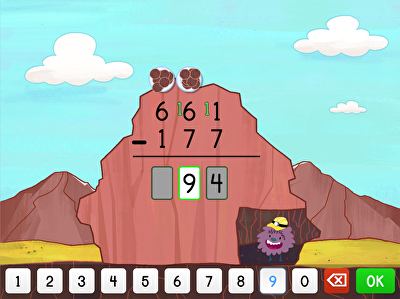In this three-digit subtraction with borrowing mountain game, students help Cuz-Cuz subtract his way through the mountain to fill his belly. Students are prompted to enter the difference on these three-digit subtraction problems one digit at a time, and to regroup by hitting the plate above the number they need to borrow from. This engaging maths game is designed with a year four audience in mind.

## Three-Digit Subtraction and Regrouping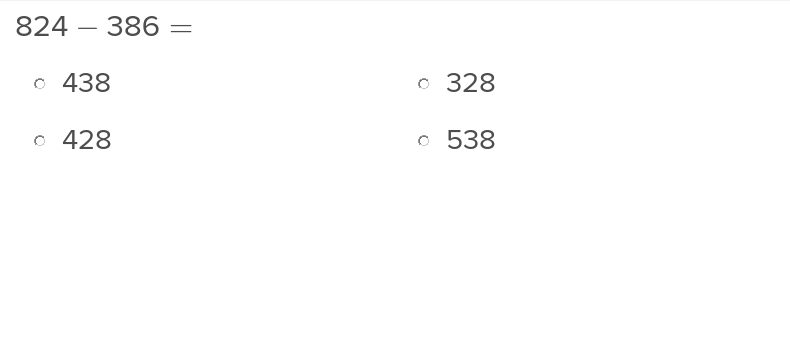Teach students how to regroup, or borrow, during three digit subtraction problems with this easy to navigate exercise.

## Three-Digit Subtraction and Regrouping Numbers with Two Zeros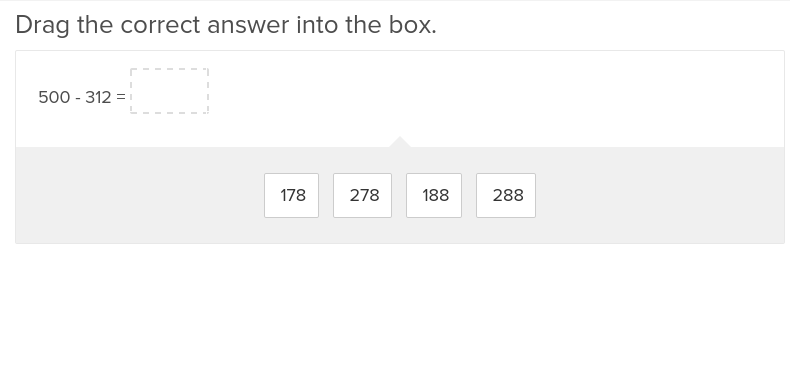When students move on to three digit subtraction, they’ll appreciate the helping hand from this exercise that shows how to regroup numbers with two zeros.

## Three Digit Subtraction and Missing Factors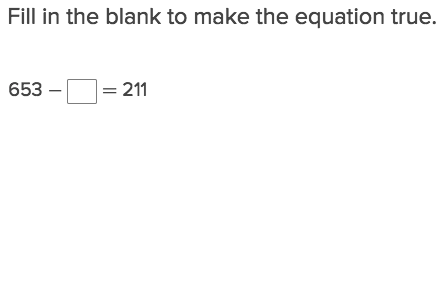If students are confused about how to complete three digit subtraction problems with missing factors, let them work through this explanatory exercise.

## Three-Digit Subtraction Word Problems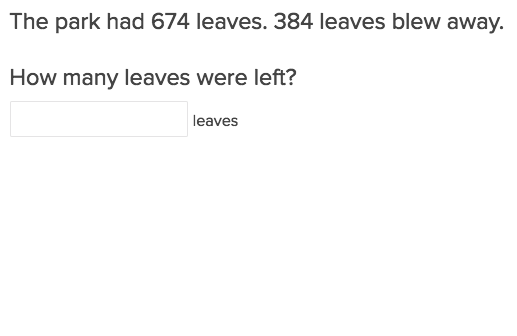This exercise will show students how to execute three digit subtraction after reading through a word problem.

## Three-Digit Subtraction and Two-Step Word Problems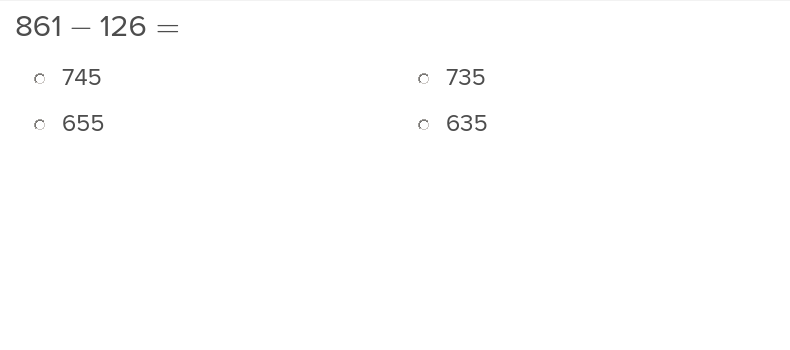This exercise shows students how to simplify complex two-step word problems that involve three-digit subtraction.

Create new collection

0

### New Collection>

0Items

What could we do to improve Education.com?